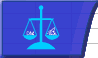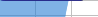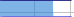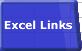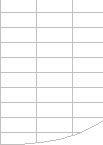# Controlling Calculation from VBASmart Recalculation Evaluation Circumstances Calculation Process Dependency Trees Controlling Calculation "CALCULATE" in Status Bar Calculation Methods Calculating from VBA Volatile Functions & Actions User-Defined Functions Repetitive Calculation Version Timing Comparison### Controlling Calculation from VBA.

For information on what the calculation methods do see Calculation Methods.

VBA allows you to control Calculation methods, properties and events:

### Calculation Methods:

#### F9 - Recalculate

Application.Calculate

#### CTRL/ALT/F9  Full Calculation

In Excel 2000 and later versions:

Application.CalculateFull

In Excel 97 there are two possible methods using either SendKeys or EnableCalculation:

You can use SendKeys to send the CTRL/ALT/F9 key sequence. This can be tricky because Sendkeys just sends the keystrokes into the keyboard buffer, and they are not normally processed until VBA has ended and you cannot guarantee that they will be processed by the correct window/application etc.

With Windows 95/98/ME:

SendKeys "%^{F9}", True
• Use Sendkeys rather than Application SendKeys.
• The True argument causes VBA to wait for the calculation to proceed without interruption. This is required unless the Sendkeys statement is that last VBA statement to be executed other than End.
• The True argument does not work with Application.SendKeys

With Windows NT/2000/XP:

Application.SendKeys "%^{F9}"
DoEvents

If the VBA procedure containing the Sendkeys statement is called directly or indirectly from a command button the True argument does not work, so you have to use DoEvents to get Win Xp and Excel97 to process them.

Another method uses the worksheet.enablecalculation property. When this property is changed all the formulae on the worksheet are flagged as uncalculated, so toggling the property to false and then back to true for all sheets in all open workbooks will cause the next calculation to be a full calculation.

Dim oSht as worksheet
Application.Calculation=xlCalculationManual

for each oSht in Worksheets
oSht.enablecalculation=false
osht.enablecalculation=true
next osht

Application.calculate

You can also use this method for a single worksheet to do a full sheet calculate.

#### Full Calculation with Dependency Rebuild

In Excel 2002 and later versions: Application.CalculateFullRebuild

In prior versions of Excel you can achieve the same effect by switching to manual, replacing all equal signs in formulae with equal signs and then either switching back to automatic or doing a manual full calculation (Ctrl/Alt/F9)

#### Shift F9  Sheet Recalculate

Worksheets(SheetName).Calculate

#### Range Calculate: see Calculation Methods for details and limitations

Range(\$a\$3:\$Z\$9).Calculate

#### Evaluate Method:

You can use the Evaluate method of the Application, Worksheet or Chartobject to return the result of calculating a string containing Names, Ranges and/or a Formulae to VBA without altering anything on a worksheet. Evaluate also enables you to get the results of a single-cell or multi-cell array formula passed to Evaluate as a string. There are two different syntaxes for Application.Evaluate, for example as Evaluate("SUM(A1:A20") or as [SUM(A1:A20)]

The difference between using Application.Evaluate and Worksheet.Evaluate is not spelled out in Excel's help.
Application.Evaluate the string as though it was on the active sheet, but Worksheet.evaluate evaluates the string as though it was on the referenced sheet:
If Sheet1!A1 contains 'fred' and Sheet2!A1 contains 'Joe', and Sheet 1 is the active sheet then Evaluate("A1") returns 'fred' but Worksheets("Sheet2").Evaluate("A1") returns 'Joe'

#### Evaluate Method limitations:

• The string must be less than 256 characters.
• A1 style references can be evaluated in both A1 and R1C1 reference mode (Application.ReferenceStyle), but R1C1 style references can only be evaluated in R1C1 mode.
• Relative references in the string are treated as absolute, unless they are contained in defined names in which case the defined name is evaluated with respect to cell A1.
• Dates should be in USA format (Month-Day-Year).
• Evaluate always treats string formulae as array formulae.
• Evaluate will return an error value if the string formulae contains external references to closed workbooks or XLM functions.
• If the string formula is a reference to a UDF ( Evaluate("=MyUdf()") ) it seems to be evaluated twice, but if its an expression containing a UDF and you are using Worksheet.Evaluate rather than Application.Evaluate ( Activesheet.Evaluate("=0+MyUdf()") ) then it only gets evaluated once.
• If the string formula contains a reference to both a UDF and a name it will fail with an error 2029 if the name reference occurs AFTER the UDF reference:
• If fred is a named range and xyz() is a user defined VBA function then this statement returns error 2029: application.Evaluate("=xyz(b1)+fred")
• This statement returns the correct value: application.Evaluate("=fred+xyz(b1)")
• Microsoft KB article 823604 identifies this problem but does not correctly diagnose the circumstances that cause it.

You can bypass many of these limitations (at the cost of performance) by inserting the formula string into a worksheet cell and then reading the resulting cell value back into a VBA variable.

#### Evaluate error handling:

If Evaluate cannot evaluate the string it returns an error rather than raising an error, so to trap the error you need to assign the result to a variant and then check the variant using ISERROR.

Public Function EVAL(theInput As Variant) As Variant
'
' if UDF evaluate the input string as though it was on this sheet
' else evaluate for activesheet
'
Dim vEval As Variant
Application.Volatile
On Error GoTo funcfail
If not IsEmpty(theInput) then
If TypeOf Application.Caller.Parent Is Worksheet Then
vEval = Application.Caller.Parent.Evaluate(Cstr(theInput))
Else
vEval = Application.Evaluate(cstr(theInput))
End If
If IsError(vEval) Then
EVAL = CVErr(xlErrValue)
Else
EVAL = vEval
End If
End If
Exit Function
funcfail:
EVAL = CVErr(xlErrNA)
End Function

The EVAL function can be called either as a UDF or from VBA.
If the input string resolves to an array formula then EVAL will return the corresponding array of results.
EVAL must be volatile because Excel cannot detect which cells the input string argument refers to, and hence does not know when the function needs to be recalculated.
EVAL returns #Value if the string cannot be evaluated, and #N/A if the function encounters any other error.

### Properties controlling Calculation Options:

#### Application.Calculation

Can be set to xlCalculationAutomatic, xlCalculationManual or xlCalculationSemiAutomatic, (in Excel95 these were xlAutomatic etc).
Resetting calculation to xlCalculationAutomatic will trigger a recalculation.

Setting these properties sometimes fails with a 1004 error when the code is called from the event code of a Control Toolbox control. You can bypass this problem by setting the TakeFocusonClick property of the control button to false, or for other controls by preceding it by Activesheet.Activate.

#### Excel's Initial Calculation Setting

FastExcel V4 allows you to control Excel’s initial calculation sequence.

Excel sets the initial calculation mode from the first non-template, non-addin workbook opened, or created and calculated.

This means that the calculation mode setting in subsequently opened workbooks will be ignored.

If you need to override the way Excel initially sets the calculation mode you can set it yourself by creating a module in ThisWorkbook (doubleclick ThisWorkbook in the Project Explorer window in the VBE), and adding this code. This example sets calculation to Manual.

Private Sub Workbook_Open()
Application.Calculation = xlCalculationManual
End Sub

Unfortunately if calculation is set to Automatic when a workbook containing this code is opened, Excel will start the recalculation process before the Open event is executed. The only way I know of to avoid this is to open a dummy workbook with a Workbook open event which sets calculation to manual and then opens the real workbook.

#### Application.CalculateBeforeSave

True or False. If calculation is Manual and CalculateBeforeSave is true when you Save the workbook it will be recalculated.

#### Application.Iteration, MaxIterations and MaxChange

If Application.Iteration is true and the workbook contains circular references, then calculation will iterate until either the number of iterations reaches MaxIterations or the largest change in cell value in the latest iteration is less than Maxchange.

#### Workbook.PrecisionAsDisplayed

If True the workbook will be calculated using the number of decimal places in the formatting.

#### Workbook.Date1904

If true date calculations in the workbook will be based on 1904.

#### Workbook.AcceptLabelsInFormulas

If true Excel will use "Natural Language" labels in formulae. For anything other than simple models this should be turned off.

#### Worksheet.EnableCalculation; Preventing Specific Worksheets being Calculated

FastExcel Version 4 gives you an improved implementation of this facility, called Mixed Mode sheets, which allows you to control which sheets will be recalculated by which type of calculation event, and saves your settings with the workbook.

Setting the Worksheet property EnableCalculation to False will prevent Excel from including the worksheet in a recalculation. This will stop the sheet being recalculated by Automatic Recalculation, F9, Ctrl/Alt/F9 and by Sheet.Calculate, Application.Calculate, Application.CalculateFull and Application.CalculateFullRebuild. The EnableCalculation property has no effect on Range.Calculate.

If you use Range.Calculate on a formula on a worksheet that has EnableCalculation set to false then at the next recalculation Excel will recalculate any formulae on other sheets that are dependent on that formula.

Setting enablecalculation to false and then back to true will flag all the formulae on the worksheet as uncalculated. If the calculation mode is Automatic a recalculation will be triggered. All calculation methods, including Sheet.Calculate but excluding Range.Calculate, will then calculate all the formulae on the sheet. You can use this method as a way of simulating Calculatefull at worksheet rather than workbook level. Note that Sheet.Calculate will not reset the uncalculated formulae flags, so two Sheet.Calculates in succession after toggling the EnableCalculation property will both do a Full Sheet Calculate.

The property is reset to true when a workbook is opened.

### Calculation Events:

#### Application or Workbook SheetCalculate

This event occurs after any worksheet is recalculated or changed chart data is replotted.

#### Worksheet or Chart Calculate

This event occurs after the Worksheet is recalculated or the chart's changed data is replotted.

### Excel 2002/2003 Only

Excel 2002/2003 considerably enhances your ability to control calculation from VBA:

#### Adding specified cells to the calculation list

You can use Range.Dirty to add the specified cells to the list of cells requiring calculation at the next recalculation.
There is a bug in Range.Dirty. It always acts on the currently active worksheet rather than the worksheet the Range object actually refers to.

#### Checking Calculation Status

The Application.CalculationState property allows you to check if calculation has completed ( xlDone ), is pending ( xlPending) , or is in process ( xlCalculating ). The Pending state seems to correspond to the message Calculate in the statusbar: for workbooks with more than 65536 dependencies the CalculationState is always xlPending or xlCalculating.

#### Interrupting Calculation

You can control the users ability to interrupt calculation by specifying what will interrupt the calculation.

Application.CalculationInterruptKey= XlAnyKey | XLEscKey | XlNokey

You can also control error handling of the interrupt (in Excel 97 onwards) using

Application.EnableCancelKey= xlDisabled | xlErrorHandler | xlInterrupt

#### Application.CheckAbort

According to Help Application.Checkabort is supposed to stop recalculation except for a specified range. It does not do this. Apparently it throws a runtime error if there are pending abort messages in the app message queue (mouse clicks, esc key down etc). The parameter specifies whether to eat the message or leave it in the queue. The objective is to allow a a long-running VBA calculation to be interrupted in the same way as an Excel calculation.

Application.CheckAbort ([KeepAbort])

### Excel 2007 Only

You can control Excel 2007's new multithreaded calculation from VBA using Application.MultiThreadedCalculation.

#### Range Calculate and RangeCalculateRowMajorOrder

Excel 2007 has two Range calculation methods. There is no standard user interface for running these calculation methods: you must call them by using VBA or some other programming language. These methods are useful when you want to calculate only a small block of cells while leaving all other formulas unchanged.

#### Range.Calculate

Range.Calculate calculates the range one row at a time, left to right and top to bottom, and then resolves all dependencies within the range. This is the same method that Excel 2002 and Excel 2003 use, except that it has been enhanced to handle iterative calculations in manual mode.

#### Range.CalculateRowMajorOrder

Range.CalculateRowMajorOrder calculates the range one row at a time, left to right and top to bottom, but it completely ignores all dependencies. This is the same method as Microsoft Excel 97 and Excel 2000 use. Because CalculateRowMajorOrder does not try to resolve dependencies, it is usually significantly faster than Range.Calculate.

If you can ensure that any dependencies within a block of formulas always refer backward to cells to the left or above, the Range.CalculateRowMajorOrder can be the fastest calculation method in Excel on a single processor system.

#### Workbook.ForceFullCalculation

You can set a new workbook property, Workbook.ForceFullCalculation , programmatically by using the Excel object model. When this property is set to True, dependencies are not loaded at open, the dependency dirty chain is not updated, and every calculation of the workbook is a full calculation rather than a recalculation.

If you have a workbook that has so many complex dependencies that loading the dependencies at workbook open takes a long time or recalculation takes longer than full calculation, you can use this property to force Excel to skip loading the dependencies and always use full calculation. Also if making a change to the workbook takes a long time in manual mode because of the time taken to dirty all the dependencies of the cell being changed, then setting Workbook.ForceFullCalculation to True will eliminate the delay.

• Although this is a workbook property the effect is at Application level rather than workbook level.
• In Excel 2007 setting the property back to False once it has been set to True has no effect on the current Excel session.
• The property is saved and restored with the workbook.
• Once this property has been set to True 'Calculate' shows in the status bar and cannot be removed by using calculation keys such as F9.© 2001-2020 Decision Models Legal Disclaimer Privacy Statement